Thermodynamics and Propulsion

5.4 Entropy Changes in an Ideal Gas

[VW, S & B: 6.5- 6.6, 7.1]

Many aerospace applications involve flow of gases (e.g., air) and we thus examine the entropy relations for ideal gas behavior. The starting point is form (a) of the combined first and second law,For an ideal gas,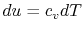. Thus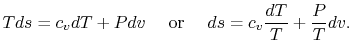Using the equation of state for an ideal gas (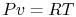), we can write the entropy change as an expression with only exact differentials:(5..2)

We can think of Equation (5.2) as relating the fractional change in temperature to the fractional change of volume, with scale factors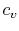and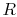; if the volume increases without a proportionate decrease in temperature (as in the case of an adiabatic free expansion), then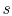increases. Integrating Equation (5.2) between two states 1'' and 2'':For a perfect gas with constant specific heatsIn non-dimensional form (using)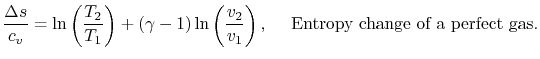(5..3)

Equation 5.3 is in terms of specific quantities. For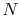moles of gas,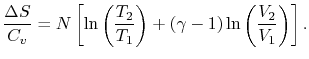This expression gives entropy change in terms of temperature and volume. We can develop an alternative form in terms of pressure and volume, which allows us to examine an assumption we have used. The ideal gas equation of state can be written as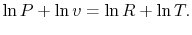Taking differentials of both sides yieldsUsing the above equation in Eq. (5.2), and making use of the relations;, we find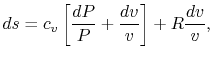or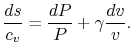Integrating between two states 1 and 2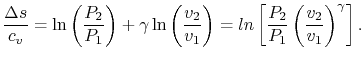(5..4)

Using both sides of (5.4) as exponents we obtain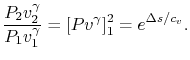(5..5)

Equation (5.5) describes a general process. For the specific situation in which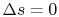, i.e., the entropy is constant, we recover the expression. It was stated that this expression applied to a reversible, adiabatic process. We now see, through use of the second law, a deeper meaning to the expression, and to the concept of a reversible adiabatic process, in that both are characteristics of a constant entropy, or isentropic, process.

Muddy Points

Why do you rewrite the entropy change in terms of? (MP 5.4)

What is the difference between isentropic and adiabatic? (MP 5.5)

Douglas Quattrochi 2006-08-06